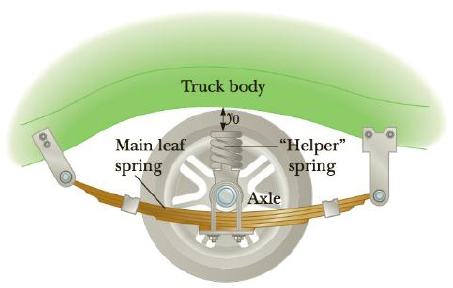Chapter 5, Problem 26P

Chapter
Section
Textbook Problem

Truck suspensions often have “helper springs” dial engage at high loads. One such arrangement is a leaf spring with a helper coil spring mounted on the axle, as shown in Figure P5.26. When the main leaf spring is compressed by distance y0, the helper spring engages and then helps to support, any additional load. Suppose the leaf spring constant is 5.25 × 105 N/m, the helper spring constant is 3.60 × 105 N/m, and y0 = 0.500 m. (a) What is the compression of the leaf spring for a load of 5.00 × 105 N? (b) How much work is done in compressing the springs?Figure P5.26

(a)

To determine
The compression of the leaf spring.

Explanation

Given Info:

The load on the suspension system is 5.0×105N .

The leaf spring constant is 5.25×105N/m .

The helper spring constant is 3.60×105N/m .

The length that the main leaf is compressed is 0.500m .

Since, when the suspension system is reached equilibrium, the total mass of the load will be equal to the total spring force acting on the load.

• Fleaf is the force on the leaf spring
• Fhelper is the force on the helper spring
• Wload is the total load on the suspension system

Let, xl is the distance that the leaf spring is compressed,

Equation (I) gives,

• kl is the spring constant of leaf spring
• kh is the spring constant of helper spring
• xl is the length that the leaf spring is compressed
• y0 is the length that the main spring is compressed

On re-arranging,

Substitute 5

(b)

To determine
The total work done for compressing the springs.

Still sussing out bartleby?

Check out a sample textbook solution.

See a sample solution

The Solution to Your Study Problems

Bartleby provides explanations to thousands of textbook problems written by our experts, many with advanced degrees!

Get Started